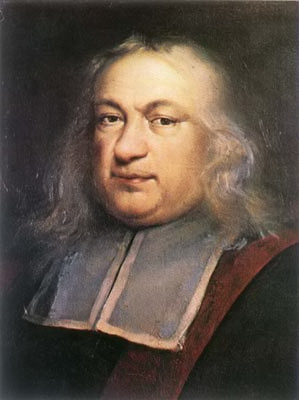## Weekly outline

•#NUMBER THEORY (MEM204) - Spring Semester 2019-20

## Department of Mathematics and Applied Mathematics - University of Crete

NOTE TO ALL STUDENTS: Please enroll so you will be automatically be updated for any news and, for any question or comment, do not hesitate to use the forum below

### Basic Information

Lecturer: Giorgos Kapetanakis (gnkapet@gmail.com) Wednesday and Friday 15.00-17.00 (Α214) Wednesday and Friday 14.00-15.00 (Γ212 Zoom link - Password: 2RcQwW) Please contact me via email if you want to arrange a meeting. Final Exam.

### Bibliography

1. Ι. Αντωνιάδης και Α. Κοντογεώργης, Θεωρία αριθμών και εφαρμογές. Εκδόσεις Κάλλιπος, 2015.
2. Μ. Παπαδημητράκης, Θεωρία Αριθμών: Πρόχειρες Σημειώσεις.
3. Ν. Τζανάκης, Θεμελιώδης Θεωρία Αριθμών.
4. Δ. Πουλάκης, Θεωρία αριθμών, εκδόσεις Ζήτη, 1997.
5. T. Apostol, Εισαγωγή στην αναλυτική θεωρία των αριθμών, Gutenberg, 2005. (μετάφραση Α. Ζαχαρίου και Ε. Ζαχαρίου)
6. K. Rosen, Elementary Number Theory and Its Applications [6th edition], Pearson, 2011.
••### 10 February - 16 February

Divisibility, Euclidean division, greatest common divisor, Euclidean algorithm, least common multiple, prime numbers (definition, basic properties, Euclid's theorem), the fundamental theorem of arithmetic. (Paragraphs 2.1-2.7 of )

•### 17 February - 23 February

We applied the fundamental theorem of arithmetic on divisibility, the greatest common divisor and the least common multiple. We defined arithmetic functions and the Dirichlet product and saw some of its basic properties. We saw some relevant examples and exercises. (Paragraphs 2.8 and 3.1 of )

•### 24 February - 1 March

We defined (completely) additive and (completely) multiplicative functions. We then focused on multiplicative functions and proved some of their properties. We then focused on the Möbius function, we proved the Möbius inversion formula and we saw the basic properties of Euler's $$\phi$$ function. We concluded with some remarks on perfect numbers. (Paragraphs 3.2, 3.4, 3.5 and 3.6 of )

•### 2 March - 8 March

We saw some exercises on arithmetic functions and answered those of the 1st set.

•### 9 March - 15 March

We defined and saw the basic properties of the integers modulo $$n$$. We defined the complete and the reduced residue systems modulo $$n$$. We proved Wilson's theorem, Euler's theorem and Fermat's little theorem. We defined the order of an integer modulo $$n$$ and saw a few relevant exercises. (Paragraphs 4.1-4.6 of )

•### 16 March - 22 March

Coronavirus won Number Theory this week...

•### 23 March - 29 March

Coronavirus won Number Theory this week too...

••### 6 April - 12 April

Linear congruences and systems of linear congruences. The Chinese Remainder Theorem. We saw a few relevant examples. (Paragraphs 4.7 and 4.8 of )

•### 13 April - 19 April

We solved the exercises of the 3rd set and saw a few additional examples. We introduced polynomial congruences. (Paragraphs 5.1-5.3 of )

•### 20 April - 26 April

Happy Easter!

•### 27 April - 3 May

Polynomial congruences modulo a prime power. Quadratic residues and the Legendre symbol. The Legendre symbol of -1 and 2, The quadratic reciprocity law. (Paragraphs 5.3, 6.1 and 6.2 of )

•### 4 May - 10 May

The Jacobi symbol and relevant examples. Further,  we answered the 4th set and we saw a few additional exercises. (Paragraph 6.3 of )

•### 11 May - 17 May

We showed that primitive roots modulo $$n$$ exist if and only if $$n=2,4,p^r$$ or $$2p^r$$, where $$p$$ is an odd prime. Also, we counted the number of these roots (if they exist). We saw the RSA cryptosystem. (Paragraph 5.4 of )

••### 25 May - 31 May

The equation $$ax^2 + by^2 + cz^2=0$$, Legendre's theorem. We answered the 5th set. (Paragraph 8.5 of )

•